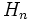# APS of groups

This term is related to: APS theory
View other terms related to APS theory | View facts related to APS theory

BEWARE! This term is nonstandard and is being used locally within the wiki. [SHOW MORE]

## Definition

An APS of groups is an APS over the category of groups. More specifically, an APS$(G,\Phi)$ of groups is the following data:

• For each natural number$n$, a group, denoted$G_n$.
• For each ordered pair$(m,n)$ of natural numbers, a homomorphism$\Phi_{m,n}:G_m \times G_n \to G_{m+n}$.

Satisfying the following compatibility conditions:

For$g, h, k$ in$G_m, G_n, G_p$ respectively,$\Phi_{m+n,p} (\Phi_{m,n}(g,h),k) = \Phi_{m,n+p} (g, \Phi_{n,p}(h,k))$.

The above condition is termed an associativity condition.

We may assume$G_0$ as the trivial group and define$\Phi_{m,0}$ and$\Phi_{0,n}$ as trivial paddings.

## Terminology

### Members and elements

For an APS$(G,\Phi)$ the member$G_n$ is termed the$n^{th}$ member of the APS. A member of the APS is an object that is the$n^{th}$ member for some$n$.

An element of the APS is an element of some member of the APS.

The home of an element of the APS is the member in which it lies. The index of a member is the$n$ for which it is the$n^{th}$ member.

### Block concatenation map

The maps$\Phi_{m,n}$ are termed block concatenation maps.

### Ground member

The ground member of an APS is its first member.

## Other notions

### Homomorphism of APSes

Further information: APS homomorphism

Given APSes$(G,\Phi)$ and$(H,\Psi)$, a homomorphism$h: G$$H$ associates, to each natural number$n$, a map$h_n: G_n$$H_n$, such that:$\Psi_{m,n}(h_m(a),h_n(b)) = h_{m+n}(\Phi_{m,n}(a,b))$

### Sub-APS notion

Further information: sub-APS

Given an APS$(G,\Phi)$, a sub-APS$H$ associates, to each$n$, a subgroup$H_n$ of$G_n$, such that the image of$H_m X H_n$ under$\Phi_{m,n}$ lies inside$H_{m+n}$.

When the APS of groups is injective, any sub-APS is also injective.

### Quotient APS notion

Further information: quotient APS

A quotient APS is the image of an APS in a homomorphism that is surjective at each member.

### Normal sub-APSes, kernels and images

Given a homomorphism of APSes of groups, the kernels of the individual homomorphisms for a sub-APS of the domain APS, and the images of the individual homomorphism form a sub-APS of the range APS. The image is thus a quotient APS.

Further, we have the following result: a sub-APS of an APS of groups occurs as the kernel of an APS homomorphism if and only if every member of it is a normal subgroup of the corresponding member of the whole APS. A sub-APS satisfying either of these equivalent conditions is termed a normal sub-APS.

This parallels the group theory result that a subgroup of a group occurs as the kernel of a group homomorphism if and only if it is normal.

## Properties

### Injectivity

An APS of groups is termed injective, or an IAPS of groups, if every block concatenation map is injective. For an IAPS of groups, we usually also assume the condition of refinability.

### Commutativity

Very few APSes of groups are commutative. Note that a commutative APS cannot also be injective.